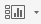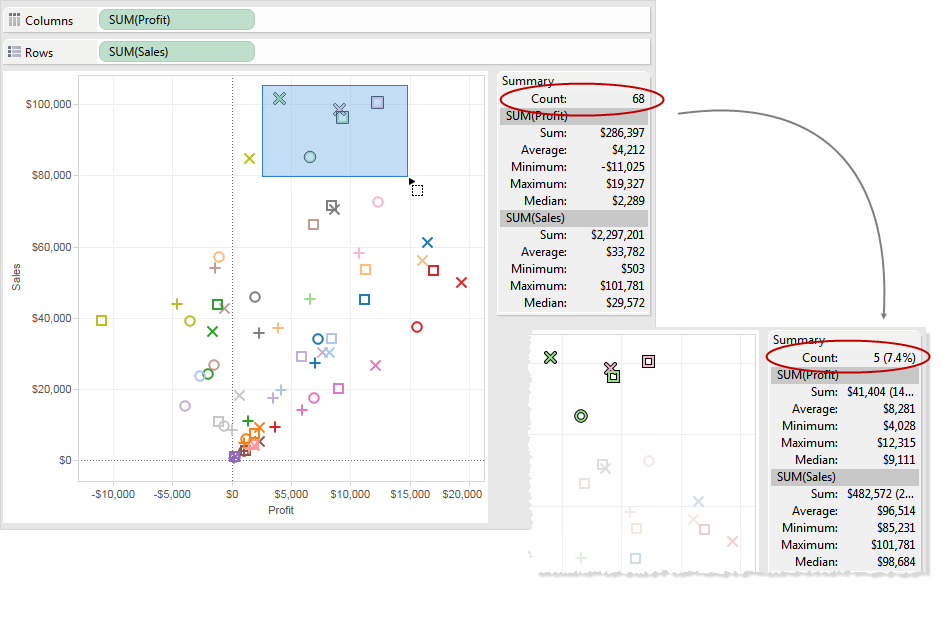# Summary Card

The Summary Card, available on the Show/Hide Cards toolbar menu, provides a quick view of information about a selection or the entire data source.

When you select data in the view, the Summary Card updates to show you information only for the data within the selection:By default, the Summary Card shows Sum, Average, Minimum, Maximum and Median values for the data in the view. The summary card values update to show these values for the current selection of data points. (Average is computed by summing all relevant values and then dividing by the total number of values. Median is computed by sorting values from lowest to highest and then selecting the middle value.) The Count value at the top of the card indicates the number of marks in the view or selection.

You can use the drop-down menu for the Summary Card to show additional statistics:

• Standard Deviation

A measure of data spread around its average, measured in the same units as the data itself. The sample standard deviation is an unbiased estimate of the population standard deviation given a slight correction. This standard deviation includes the correction.

• First Quartile

A measure of location that is commonly used with other quartiles to provide a robust measure of spread. Robust in this case means not as sensitive to outliers as the standard deviation. The first quartile is the 25th percentile, typically the lower line in a boxplot.

• Third Quartile

A measure of location that is commonly used with other quartiles to provide a robust measure of spread. Robust in this case means not as sensitive to outliers as the standard deviation. The third quartile is the 75th percentile, typically the upper line in a boxplot.

• Skewness

A measure of the tendency of your data to have extreme values to one side. Positive skewness means the extreme values are to the right, while negative skewness means the extreme values are to the left.

• Excess Kurtosis

A measure of the tendency of your data to have more extreme or outlying values than a normal distribution. A normal distribution has a kurtosis of 3 so this value is kurtosis minus three.